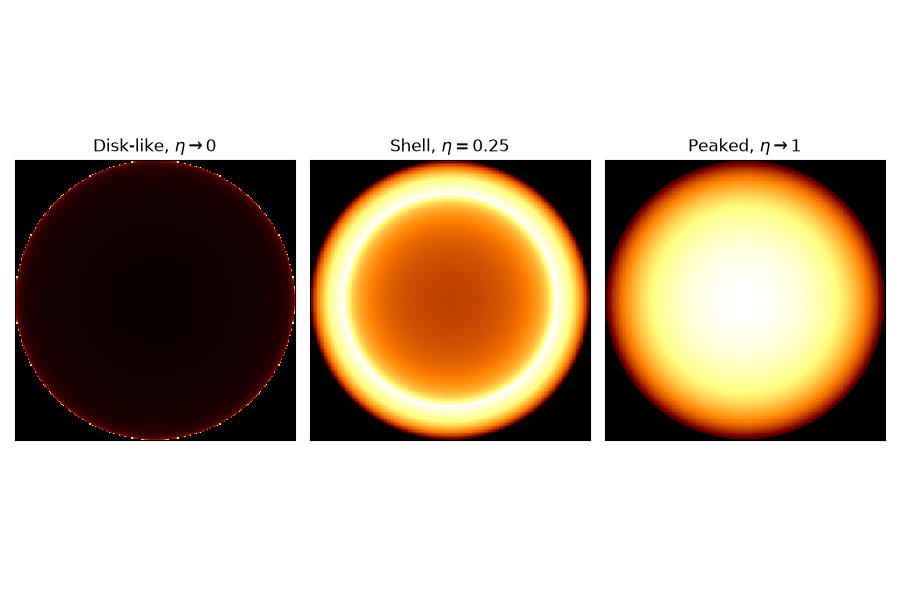Shell2 spatial model¶

This is a spatial model parametrizing a projected radiating shell.

The shell spatial model is defined by the following equations:

$\begin{split}\phi(lon, lat) = \frac{3}{2 \pi (r_{out}^3 - r_{in}^3)} \cdot \begin{cases} \sqrt{r_{out}^2 - \theta^2} - \sqrt{r_{in}^2 - \theta^2} & \text{for } \theta \lt r_{in} \\ \sqrt{r_{out}^2 - \theta^2} & \text{for } r_{in} \leq \theta \lt r_{out} \\ 0 & \text{for } \theta > r_{out} \end{cases}\end{split}$

where $$\theta$$ is the sky separation, $$r_{\text{out}}$$ is the outer radius and $$r_{\text{in}}$$ is the inner radius.

For Shell2SpatialModel, the radius parameter r_0 correspond to $$r_{\text{out}}$$ and the relative width parameter is given as eta = $$(r_{\text{out}} - r_{\text{in}})/r_{\text{out}}$$ so we have $$r_{\text{in}} = (1-eta) r_{\text{out}}$$.

Note that the normalization is a small angle approximation, although that approximation is still very good even for 10 deg radius shells.

Example plot¶

Here is an example plot of the shell model for the parametrization using outer radius and relative width. In this case the relative width, eta, acts as a shape parameter.

import matplotlib.pyplot as plt
from gammapy.modeling.models import (
Models,
PowerLawSpectralModel,
Shell2SpatialModel,
SkyModel,
)

tags = [
r"Disk-like, $\eta \rightarrow 0$",
r"Shell, $\eta=0.25$",
r"Peaked, $\eta\rightarrow 1$",
]
eta_range = [0.001, 0.25, 1]
fig, axes = plt.subplots(1, 3, figsize=(9, 6))
for ax, eta, tag in zip(axes, eta_range, tags):
model = Shell2SpatialModel(
lon_0="10 deg",
lat_0="20 deg",
r_0="2 deg",
eta=eta,
frame="galactic",
)
model.plot(ax=ax)
ax.set_title(tag)
ax.set_xticks([])
ax.set_yticks([])
plt.tight_layout()YAML representation¶

Here is an example YAML file using the model:

pwl = PowerLawSpectralModel()
shell2 = Shell2SpatialModel()

model = SkyModel(spectral_model=pwl, spatial_model=shell2, name="pwl-shell2-model")

models = Models([model])

print(models.to_yaml())

Out:

components:
-   name: pwl-shell2-model
type: SkyModel
spectral:
type: PowerLawSpectralModel
parameters:
-   name: index
value: 2.0
-   name: amplitude
value: 1.0e-12
unit: cm-2 s-1 TeV-1
-   name: reference
value: 1.0
unit: TeV
frozen: true
spatial:
type: Shell2SpatialModel
frame: icrs
parameters:
-   name: lon_0
value: 0.0
unit: deg
-   name: lat_0
value: 0.0
unit: deg
-   name: r_0
value: 1.0
unit: deg
-   name: eta
value: 0.2

Gallery generated by Sphinx-Gallery